# Calculus 3 : Double Integration over General Regions

## Example Questions

1 3 Next →

### Example Question #686 : Multiple Integration

Calculate the following Integral.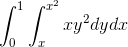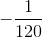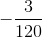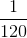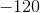Explanation: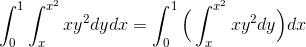Lets deal with the inner integral first.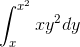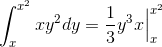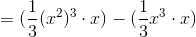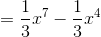Now we evaluate this expression in the outer integral.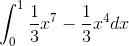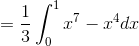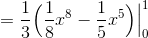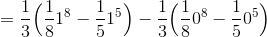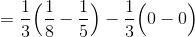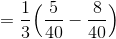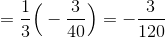### Example Question #687 : Multiple Integration

Calculate the following Integral.Explanation:Lets deal with the inner integral first.Now we evaluate this expression in the outer integral.1 3 Next →

### All Calculus 3 Resources